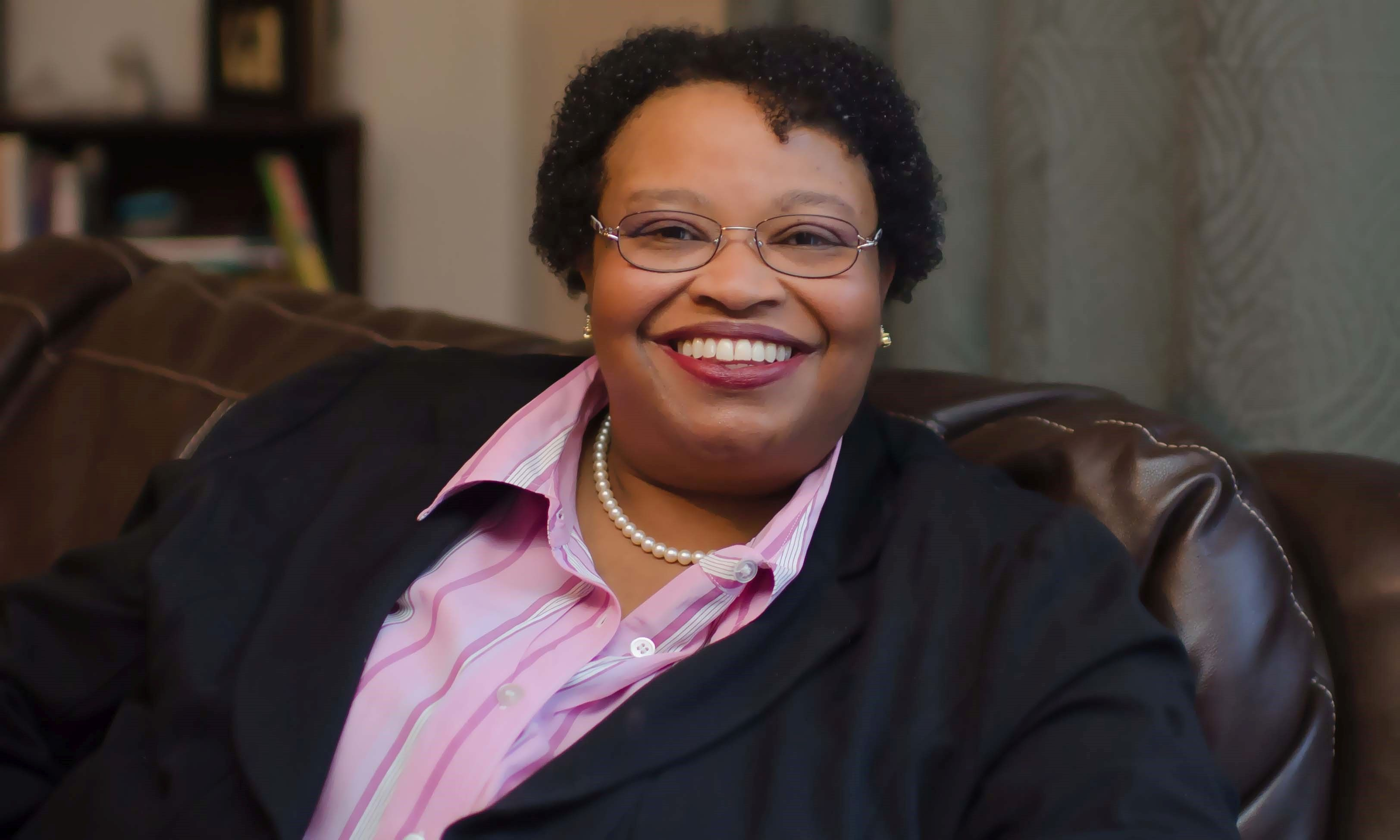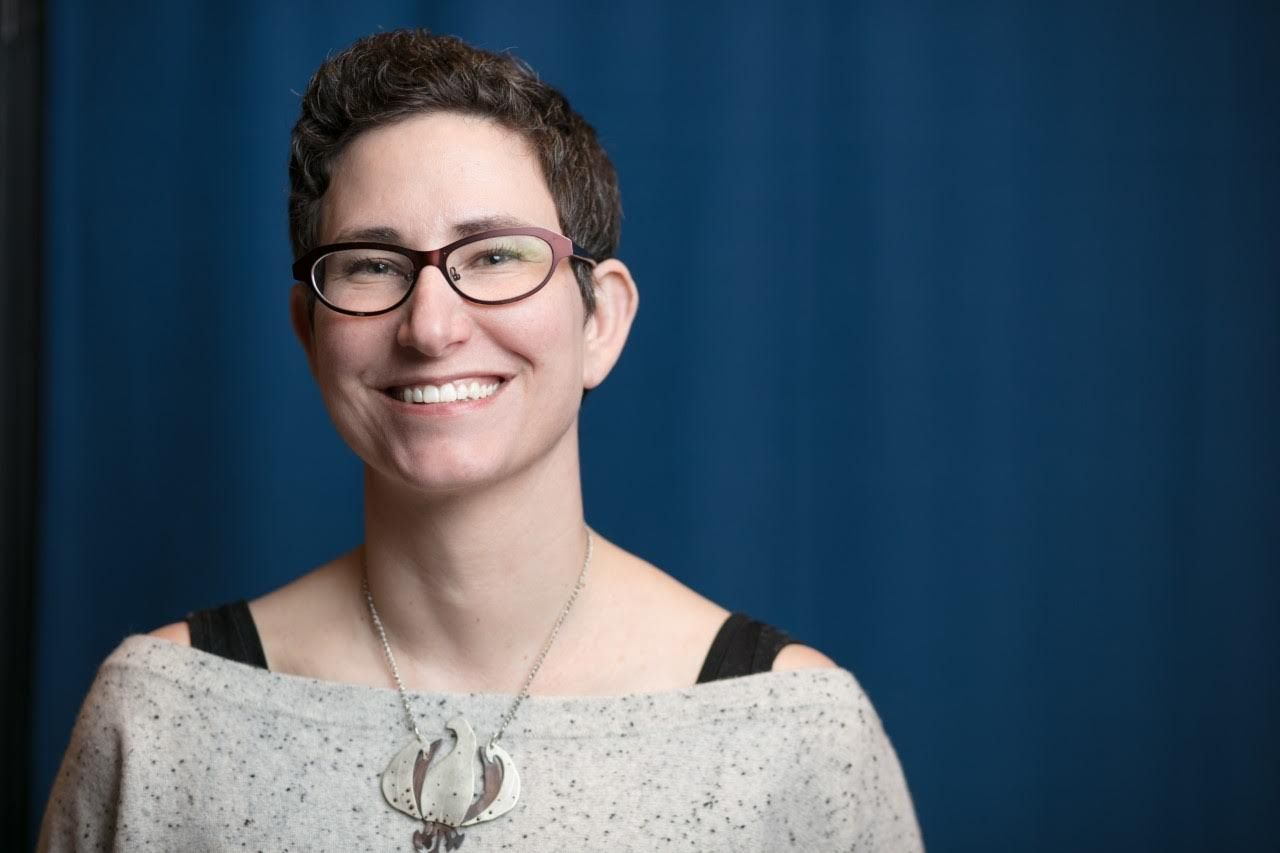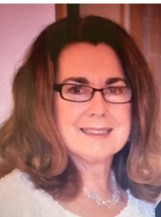The Federation is the oldest and only Jewish Women's
Federation in the Country - Since 1916

*ALL MEMBERS OF OUR CONSTITUENT ORGANIZATIONS

ARE MEMBERS OF FEDERATION

<<<<<<<<<<<<<<<<<<<<<<<<<<<<<<<<<<<<<<<<<<<<<<<<<<<<<<<<<<<Visit and Like us on Facebook to Stay Informed!

<<<<<<<<<<<<<<<<<<<<<<<<<<<<<<<<<<<<<<<<<<<<<<<<<<<<<<<<<<<

OPENING MEETING
Thursday, September 15, 2022
9:30am Virtual

"Envisioning a Truly Inclusive Jewish Community"

Guest SpeakersDr. Harriette E. Wimms

andTracie Guy-Decker

Registration deadline, Friday, September 9, 2022
MORE MEETING INFO TO COME.....

<<<<<<<<<<<<<<<<<<<<<<<<<<<<<<<<<<<<<<<<<<<<<<<<<<<<<
What is Serv-A?

<<<<<<<<<<<<<<<<<<<<<<<<<<<<<<<<<<<<<<<<<<<<<<<<<<<<<

President, CeCe Rund<<<<<<<<<<<<<<<<<<<<<<<<<<<<<<<<<<<<<<<<<<<<<<<<<<<<<

Slate of Officers for 2022-2023
President - CeCe Rund
Vice President - Julie Bernstein
Vice President - Shulamit Finkelstein
Vice President - Edie Meyers
Recording Secretary - Sylvia Katzel
Corresponding Secretaries - Rochelle Bohrer, Ferne Rogow
Treasurer - Ann Fishkin
Auditor - Rosalind Asch
Community Representative - Marcia Bornfirend, Past President

<<<<<<<<<<<<<<<<<<<<<<<<<<<<<<<<<<<<<<<<<<<<<<<<<<<<<

 The Federation of Jewish Women's Organizations of Maryland is the Oldest Jewish Women's Federation in the Country Founded 1916Calendar To see the full calendar and Jewish holidays, CLICK HERE.